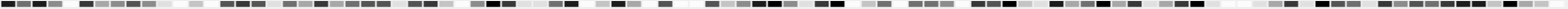# INTRODUCTION

## Is Pi normal?

Pi is a surprising number.
Over hundreds of years mathematicians have found dozens of presentations and armies of formulas to compute the value of Ludolph's number. Regardless of this fact any new record in Pi calculation demonstrates the randomness of the digit series. Frequency analysis of these results didn't point out any regularity in these sequences.
So, how can welldefinied formulas produce random output?
David Bailey and Richard Crandall are working on a proof that the famous BBP-formula for the hexadecimal presentation of a certain PI-digit generates randomizes digits.

The exact mathematical proof for Pi's "randomness" ist not given yet. Thus any deeper statistical examination of known PI sequences could be helpful. The results of those tests so far are suprisingly poor. Most of them present even digit counts. Others are based on a small data material only.
Nowadays an ordinary home pc can manage huge raw material. And since I am “Pi-minded” for a long time (see also MAGIC PIWORLD) the decision was made to set my computer on the trail. Results presented below are derived with a self-developed statistical program named PiCollector.

## AN ILLUSTRATION

Image shows the first 100x100 = 10.000 digits of Pi. Shades of gray are choosen from light ("0") to dark ("9").
Is this a random pattern?## GOALS / BASIC RESULT

The goal of this project was to proof if

1.  Pi is “NORMAL”
2.  The subsequences of the decimal presetation of Pi are IID (independently and identically distributed uniform draws)
3.  The decimal presentation of the number Pi is “RANDOM”

For estimating the various test results Chi square value was calculated first and compared with the critical Chi2 value on a given significance level (typically 99,5%).
Test with suspicious Chi2 values were proofed by the number of series differing more than 1, 2 or 3 sigma from mean value.

Any counterexmaple againts a., b. or c. would be of great interest.
Unsurprisingly, not any was found.

## DATA

In 2003 I executed a couple of statistical tests with 4.200.000.000 decimal digits of the number Pi.
All details, results and background information where exposed here:   Pi Statistics for 4,2 x 109 decimal digits.
Reading the announcment of a new calculation record by Fabrice Bellard in december 2009 the decision was made to extend the analysis to more digits. Fabrice handed me over the necessary digits  -Thanks a lot!- and the project started.

## SOFTWARE / HARDWARE

All test where done using my own “Pi-Collector” Software developed with Delphi.
Program was executed by an ordinary dual-core Pentium Windwos PC and some of the caculations took more than a day.
That’s one of the reasons why I stopped at 100.000.000.000 ;-)